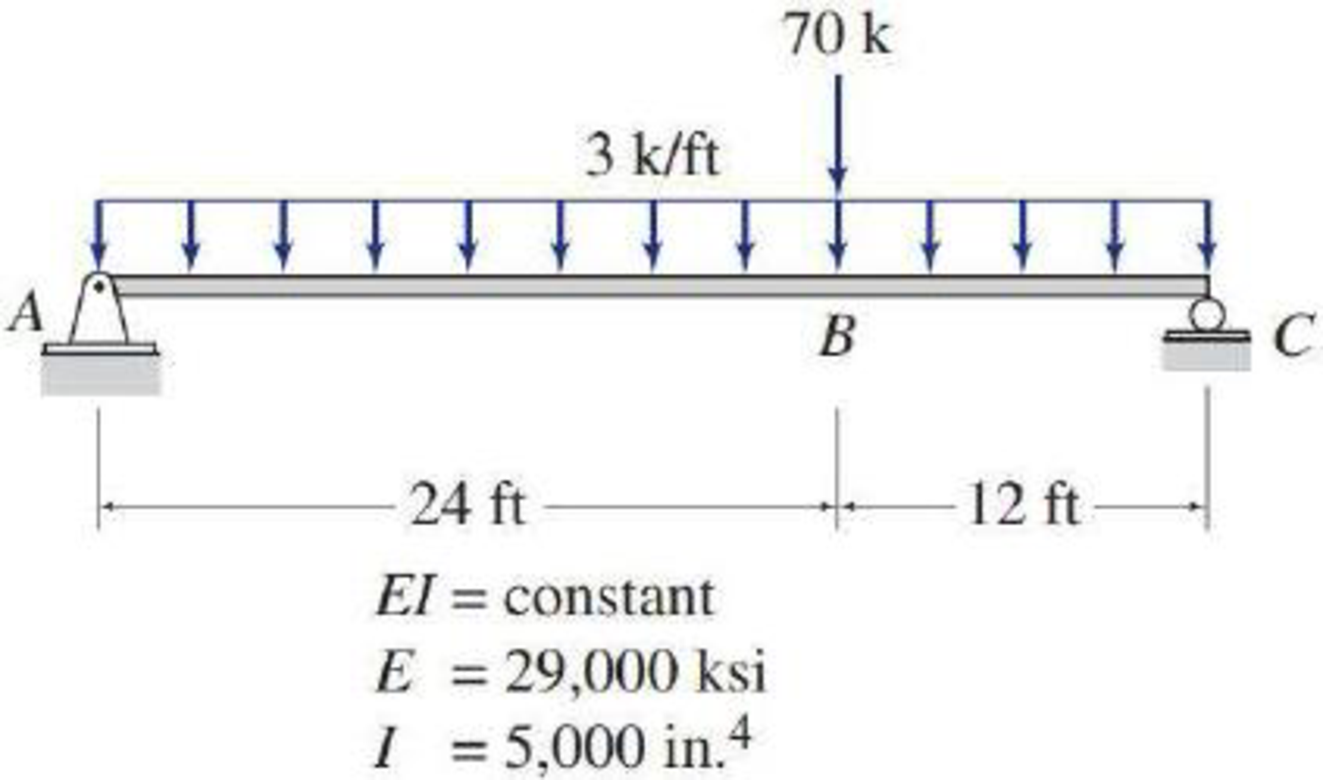# 6.23 through 6.30 Determine the maximum deflection for the beam shown by the moment-area method. FIG. P6.28, P6.54

#### Solutions

Chapter
Section
Chapter 6, Problem 28P
Textbook Problem
380 views

## 6.23 through 6.30 Determine the maximum deflection for the beam shown by the moment-area method.FIG. P6.28, P6.54To determine

Find the maximum deflection Δmax for the given beam by using moment-area method.

### Explanation of Solution

Given information:

The Young’s modulus (E) is 29,000 ksi.

The moment of inertia (I) is 5,000in.4.

Calculation:

Consider rigidity modulus (EI) of the beam is constant.

Show the free body diagram of the given beam as in Figure (1).

The given beam carries uniformly distributed load and a point load. The loading of a beam has to be divided into carrying a point load and uniformly distributed load respectively to draw the M/EI diagram.

Consider upward is positive and downward is negative.

Consider clockwise is negative and counterclockwise is positive.

Determine the reaction at A using the relation;

MC=0RA×36(3×36×362)=0RA=1,94436RA=54kips

Determine the support reaction at C using the relation;

V=0RA+RC(3×36)=0RC=10854RC=54kips

Show the reactions of the given beam as in Figure (2).

Determine the bending moment at A;

MA=(54×36)(3×36×362)=0

Determine the bending moment at center of the beam;

Mcenter=(3×18×182)+(54×18)=486+972=486kips-ft

Determine the bending moment at C;

MC=(54×36)(3×36×362)=1,9441,944=0

Show the M/EI diagram (uniformly distributed load) for the given beam as in Figure (3).

Show the free body diagram of the given beam as in Figure (4).

Determine the reaction at A using the relation;

MC=0RA×36(70×12)=0RA=84036RA=23.333kips

Determine the support reaction at C using the relation;

V=0RA+RC70=0RC=7023.333RC=46.667kips

Show the reactions as in Figure (5).

Determine the bending moment at A;

MA=(46.667×36)(70×24)=1,6801,680=0

Determine the bending moment at B;

MB=(23.333×24)=560kips-ft

Determine the bending moment at C;

MC=(23.333×36)(70×12)=840840=0

Show the M/EI diagram (point load) for the given beam as in Figure (6).

Show the elastic curve diagram as in Figure (7).

Refer Figure (3) and (6),

Determine the deflection between C and A using the relation;

Divide the M/EI into triangle and rectangle to evaluate the deflection.

ΔCA=[MEI(Areaofparabola)+MEI(Areaoftriangle)+MEI(Areaoftriangle)]

Substitute 486EI for MEI, (23×36×(362)) for area of parabola, (560EI) for MEI, (12×24×(13×24+12)) for area of triangle, 560EI for MEI, and (12×12×(23×12)) for area of triangle.

ΔCA=[486EI(23×36×(362))+(560EI)(12×24×(13×24+12))+560EI(12×12×(23×12))]=1EI[209,952+134,400+26,880]=371,232kips-ft3EI

Determine the slope at A using the relation;

θA=ΔCAL

Substitute 371,232kips-ft3EI for ΔBD and 36 ft for L

### Still sussing out bartleby?

Check out a sample textbook solution.

See a sample solution

#### The Solution to Your Study Problems

Bartleby provides explanations to thousands of textbook problems written by our experts, many with advanced degrees!

Get Started

Find more solutions based on key concepts
Determine the moment created by the weight of the traffic light about point O. The dimensions of the traffic li...

Engineering Fundamentals: An Introduction to Engineering (MindTap Course List)

Were these steps effective? Why or why not?

Fundamentals of Information Systems

What is a fully distributed database management system?

Database Systems: Design, Implementation, & Management

What is the difference between synthetic and semi-synthetic cutting fluids?

Precision Machining Technology (MindTap Course List)

Explain the activities and users involved in the detailed design phase. Define the term, prototype.

Enhanced Discovering Computers 2017 (Shelly Cashman Series) (MindTap Course List)

If your motherboard supports ECC DDR3 memory, can you substitute non-ECC DDR3 memory?

A+ Guide to Hardware (Standalone Book) (MindTap Course List)

What metals can be cut with the oxyfuel gas process?

Welding: Principles and Applications (MindTap Course List)